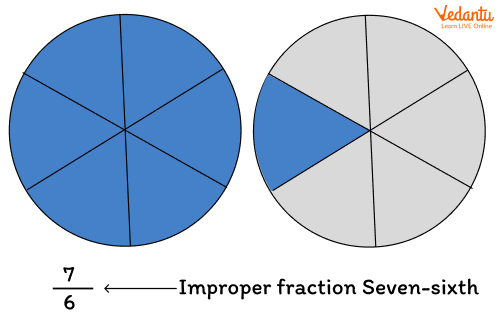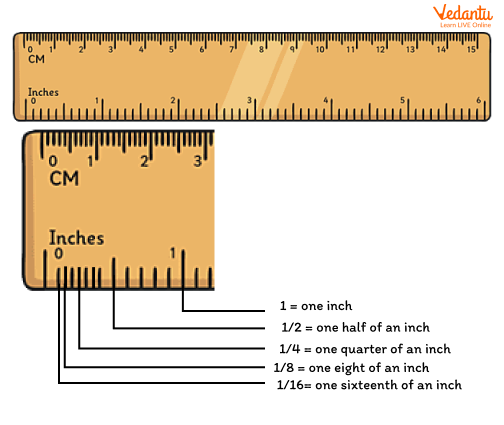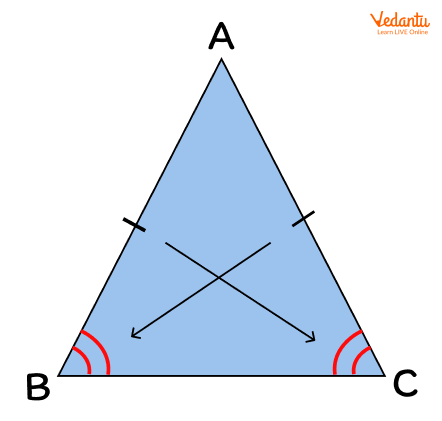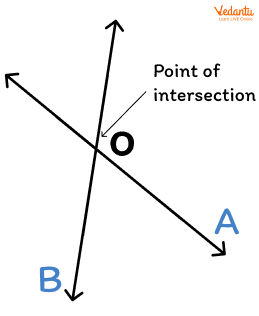Courses
Courses for Kids
Free study material
Free LIVE classes
MoreThere are a lot of terminologies that we use in Mathematics. The vocabulary of Maths is important because it helps us to know the terms and their meaning which are eventually helpful in grabbing concepts. In this article, we will discuss some of the words that start with the letter ‘I’. The list of the words is provided with a detailed explanation of each word so that students can understand the meaning of the words and use them in Maths.

## List of Words

Given below is the list of words starting with the letter ‘I’ which are most commonly used in Maths.

 S.No. Words S.No. Words 1 Improper Fraction 5 Inch 2 Inequality 6 Inverse 3 Interval 7 Isosceles Triangle 4 Is the same as 8 Intersecting Lines

Let’s know these words in detail.

1. Improper Fraction

We know that a fraction is used to represent a part of something whole of an object or number. An improper fraction is a type of fraction where the numerator is equal to or greater than the denominator. For example, 7/6 is an improper fraction. See the following representation of 7/6.1. Inequality

In Maths, inequality shows the relationship between two or sometimes more expressions that are not equal. The sign of inequality is "".

1. Interval

Interval is the opposite of continuous. Interval means that it contains gaps. We can see examples of intervals in our daily life such as the blinking of ambulance lights, blinking of the traffic lights on a regular interval, etc. In Maths, interval means the gap between time, numbers, or in any Mathematical quantity. The time interval is defined as the time gap between any two events.

1. Is the same as

It is used to show similarities between two Mathematical expressions or numbers.

1. Inch

In the customary system of measurement, an inch is a unit of length. An inch in a metric system is equal to 2.54 cm.1. Inverse

The word inverse is the opposite of another operation in Mathematics. A multiplicative inverse is a number that when multiplied by a number gives 1 as the product. If y is any non-zero number, then multiplying y by what number gives the product as 1?

• y × ? = 1

As, y/y = 1, we need such a number in which y is in the denominator’s place. We have y in the numerator. So, we keep 1 as the numerator of this number and get 1/y.

By multiplying y with 1/y, we get

• y × 1⁄y = 1

So, 1⁄y is the multiplicative inverse of y.

1. Isosceles Triangle

An isosceles triangle is a triangle having two sides of equal length. The two equal sides of this triangle are referred to as ‘legs’ and the third or unequal side is known as the ‘base’. The angles that are opposite to equal sides in such a triangle are always of equal measure. In the given figure of isosceles triangle ABC, if side AB = side AC, then angle B = angle C.1. Intersecting Lines

Intersecting lines are those lines that cross each other in a plane. The intersecting lines contain a point that used to be the common point of these lines which is known as the point of intersection. In the following image, two lines P and Q are intersecting at point O, which is the point of intersection.## Conclusion

The above article discusses all the important words that start with the letter I in Mathematics. Students must be familiar with these terms as these form the base of the students for higher classes’ Maths concepts. We hope that the article stimulates children's interest in Mathematics.

Last updated date: 29th Sep 2023
Total views: 138.6k
Views today: 2.38k